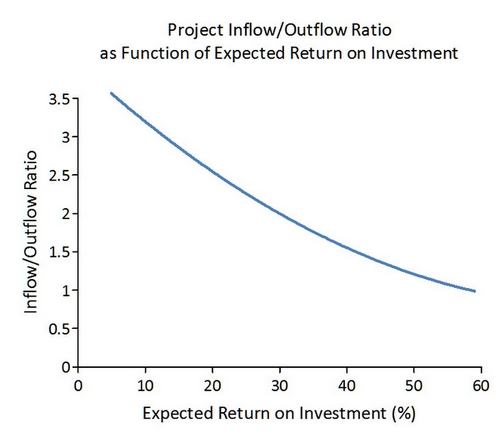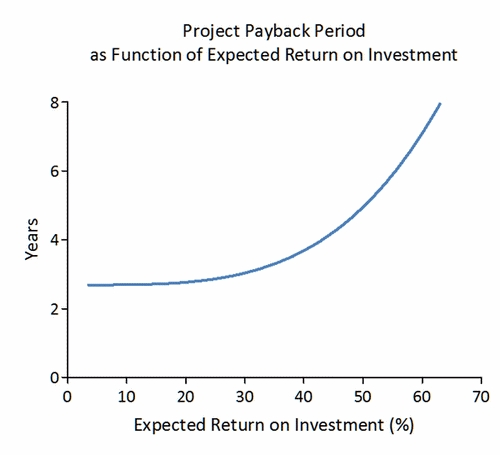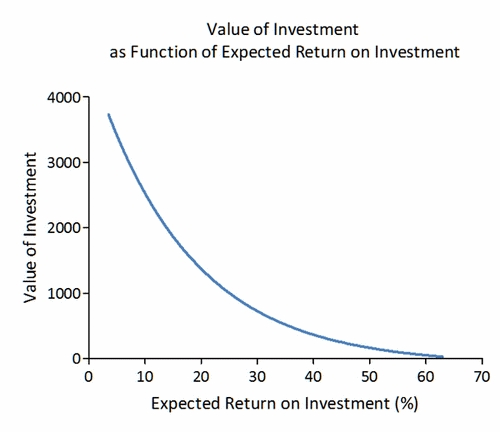# Investment analysis

This type of  analysis gives the client direct information regarding the investments profitability, and its influence on company value.

An important part of the investment analysis is cost. Especially in complex and demanding projects, there is significant risk related to investing. We have developed a method that clearly assesses and explains the relationship between uncertainty raised by each cost element, and which effect it has on the investment’s total risk.

In a complex project there is often a set of cost elements that triggers follow-up costs. If one cost element exceed budget, the total cost increase can be significant, even though the isolated increase in the original cost element seemed small.

We have focused on visualizing for the client, the scope of uncertainty in the investment, and which probability distributions that exist for each single cost element overstep.

We focus especially on those cost elements that should be subject to strong control and follow-up. Such that the likelihood for excess cost can be reduced.

We see it as crucial that the client has solid analysis describing and explaining the possible total cost outcomes, and their probability distributions at time of decision. With this method and these results the client can discuss the premises for the investment and decide whether or not carry out the investment with the revealed uncertainties.

Below we present three graphs that shows the sensitivity in a project related to changes in demand for return on capital. For all three graphs the reference point is set in the projects IRR which is the point where the projects discounted revenues equal the value of the projects discounted cost payments (Project inflow/outflow ratio = 1).The curves are ideal for comparing investments with different profiles. When the X-axes is equal, curves for different projects can be overlapped to give information on whether one project is better than the other.It is also possible to estimate the probability distribution for the projects net present value, or its effect on the distribution of company value.